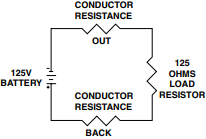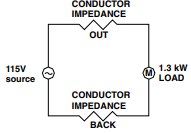Date:

# Voltage Drop Calculation for Single Phase and Three Phase SystemsElectric Panel Board

When it comes to voltage drop calculations, there are several valuable individual approach that can be used when working with electrical systems. Thus, it is important for an engineer to determine to match his calculations against the actual scenario in the type system or type of installation. The following scenario covers comprehensive calculation involving electric circuits in different scenario.

Example 1. Calculate voltage drop in a single-phase dc circuit.

A solid #14 copper two-wire cable that is 750 feet long supplies a 125 ohm load resistor for heating purposes at 75 deg. C. Find the voltage drop in the cable; and find the resulting voltage across the load resistor.Diagram: DC Circuit

Solution:

Calculate the cable resistance, for the sake of illustration, the overall length need to be multiplied by two to consider the cable resistance (out) and cable resistance (back). Assume that the cable has a uniform resistance of 75 Deg. C.

Solve for cable resistance,

Cable resistance = (RESISTANCE FOR 1000 FT.) x (750 / 1000) x 2

• R = (3.07 ohms per M ft.) X (0.750) x 2
• R = 2.3 ohms x 2
• R = 4.6 ohms.

Total resistance  = 125ohms + 2.3 ohms  = 129.6 ohms

Current = 125/ 129.6 = 0.9645 Amperes

Voltage drop in cable = current x cable resistance

• Vd = (0.9645) X (4.6)

Therefore,

• Voltage Drop in the Cable = 4.436 V
• Voltage at the load terminal = 0.9645 x 125 ohms =  120.56 Volts

Example 2. Calculate the approximate voltage drop in a single-phase ac circuit at unity power factor in a plastic conduit.

From a 115VAC circuit breaker, a solid #12 copper two-wire cable in plastic conduit that is 750 feet long supplies a motor load that requires 1.3 kW. Find the approximate voltage drop in the cable?; and find the resulting voltage supplied to the motor load? (Note: ignore the constant kVA motor action).Diagram: AC circuit

Solution:

Cable resistance = (RESISTANCE FOR 1000 FT.) x (750 / 1000) x 2

• R = (1.70 ohms per M ft.) X (0.750) x 2
• R = 1.275 ohms x 2
• R = 2.55 ohms.

Calculate the total current flowing in the circuit.

• I = 1,300/ 115 (ignoring power factor)
• I = 11.3 Ampere

Voltage drop in cable = current x cable resistance

• Vd = (11.3) X (2.55 ohms)
• Vd = 28.82 Volts

Therefore,

• Voltage Drop in the Cable = 28.82 Volts
• Voltage at the load terminal = 115 - 28.82 = 86.18 Volts

Example 3. Calculate voltage drop in a three-phase ac circuit at less than unity power factor.

From a 480/3P circuit breaker, a stranded #0000 copper three-wire Type TC (non-armored) cable that is 280 ft. long and is laid into in aluminum cable tray. The cable supplies an AC load operating at a power factor of 85% that draws 200 amperes. Find the voltage drop in the cable; and find the resulting voltage supplied to the load.Diagram: 3- phase AC circuit

Solution:

Cable resistance = (RESISTANCE FOR 1000 FT.) x (280 / 1000) - per line

• R = (0.078 ohms per M ft.) X (0.0.28)
• R = 0.0218 ohms - per line

The current is given at 200 Amperes.

Voltage drop in cable = current x cable resistance

• Vd = (200) X (0.0218 ohms) x 1.73 (multiple a factor 1.73 for 3 phase systems)
• Vd = 7.55 Volts

Therefore,

• Voltage Drop in the Cable = 7.55 Volts
• Voltage at the motor terminals = 480 - 7.55 = 472.45 Volts